1354字，阅读需时5分钟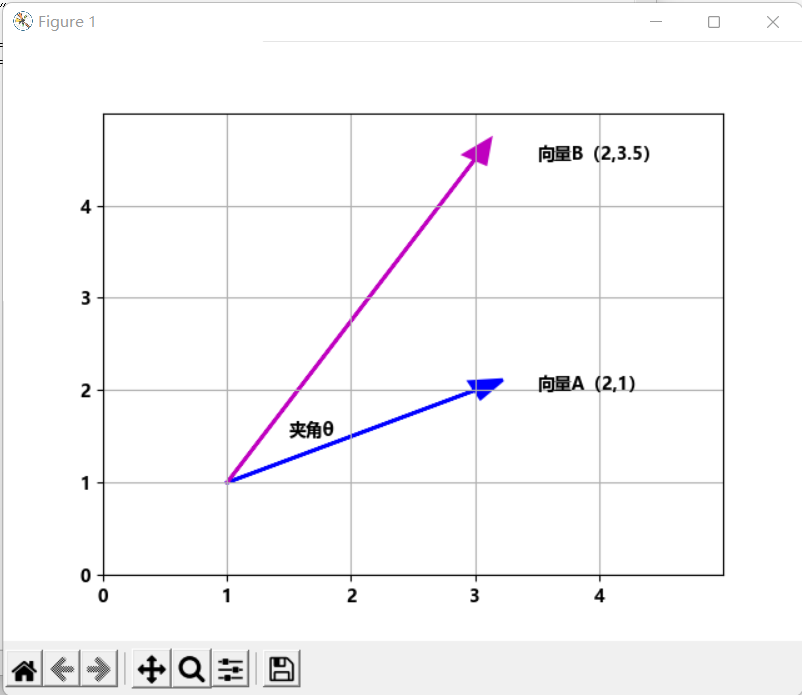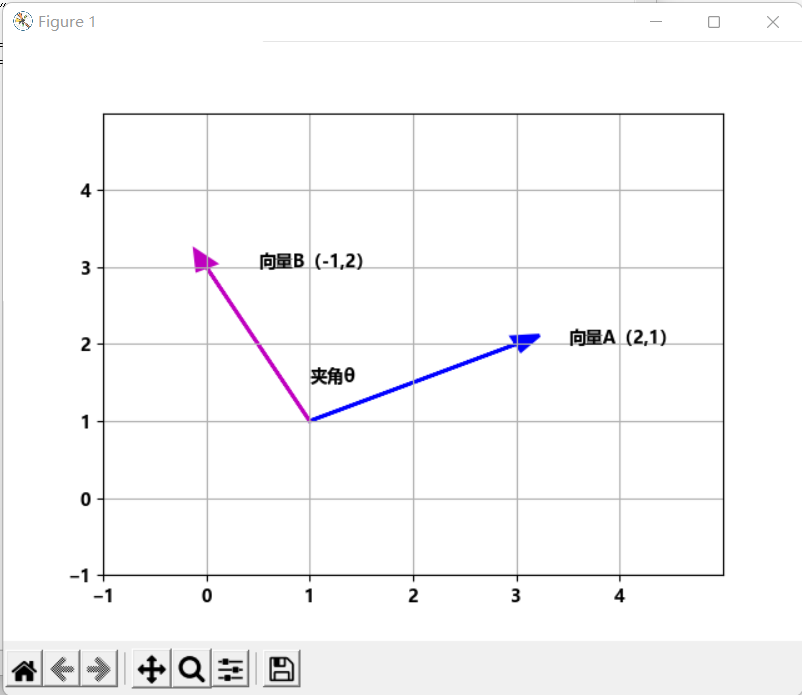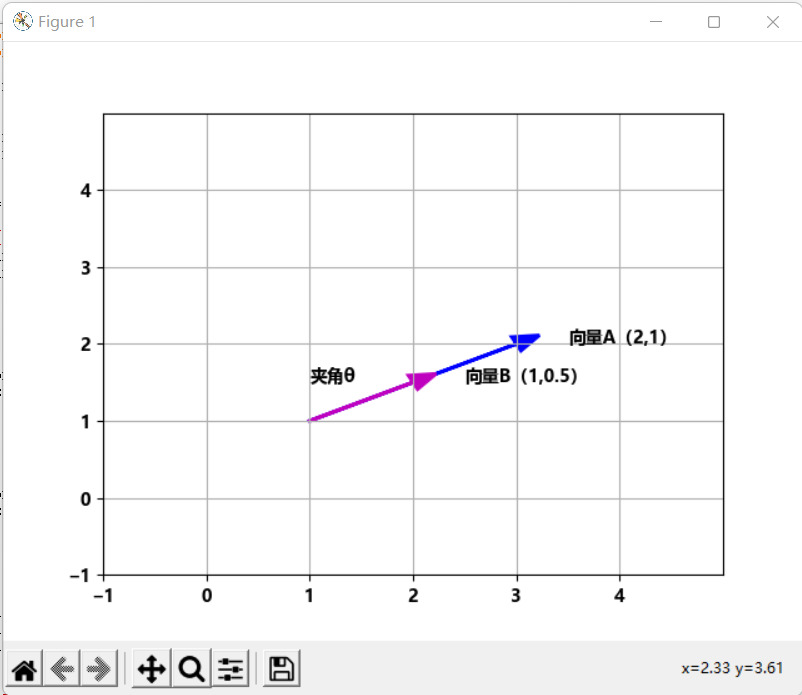import numpy as np
import matplotlib.pyplot as plt

plt.rc("font",family='MicroSoft YaHei',weight="bold")

# 定义向量A和B
A = np.array([2,1])
B = np.array([2,3.5])

# 创建坐标轴
ax = plt.axes()

#  (1,1)为起点，绘制向量A和B

# 设置X轴范围
plt.xlim(0,5)

# 设置X轴刻度
major_xticks = np.arange(0, 5)
ax.set_xticks(major_xticks)

# 设置Y轴范围
plt.ylim(0, 5)

# 设置Y轴刻度
major_yticks = np.arange(0,5)
ax.set_yticks(major_yticks)

# 绘制网格线
plt.grid(b=True, which='major')

# 绘制标注
ax.text(A+1.5,A+1,"向量A（2,1）")
ax.text(B+1.5,B+1,"向量B（2,3.5）")
ax.text(1.5,1.5,"夹角θ")

# 显示图像
plt.show()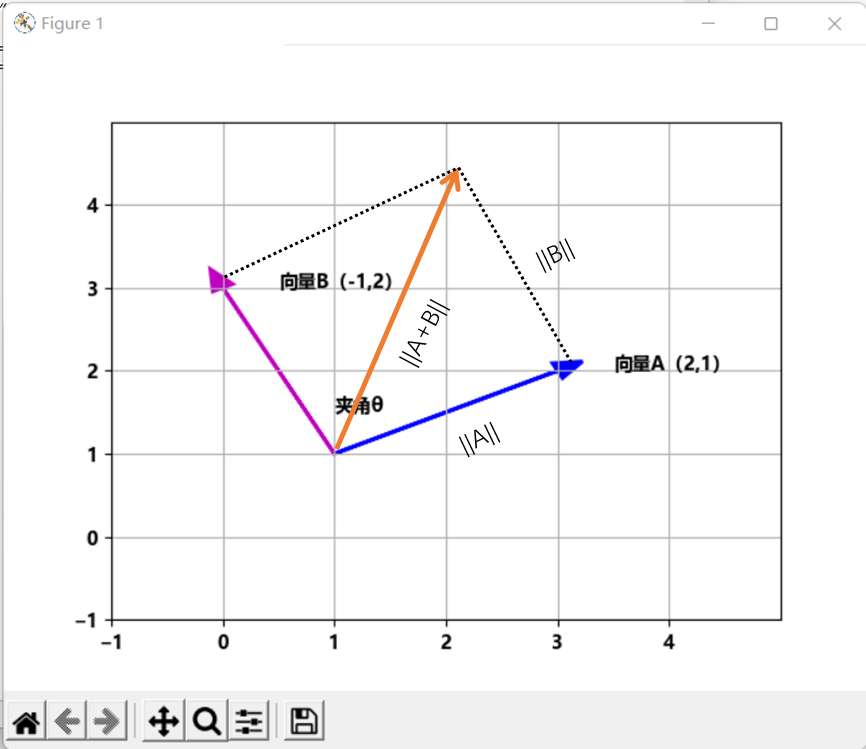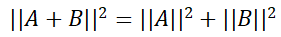||A+B||^2
=(A+B)*(A+B)
=A*A+2A*B+B*B
=||A||^2+||B||^2+2A*B